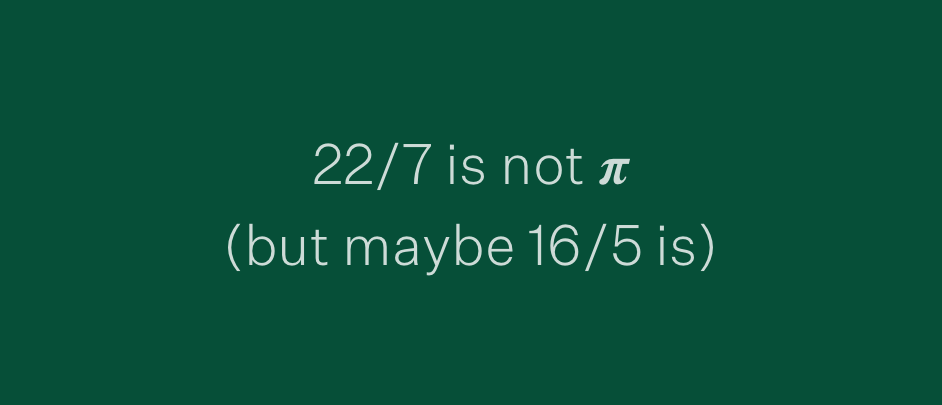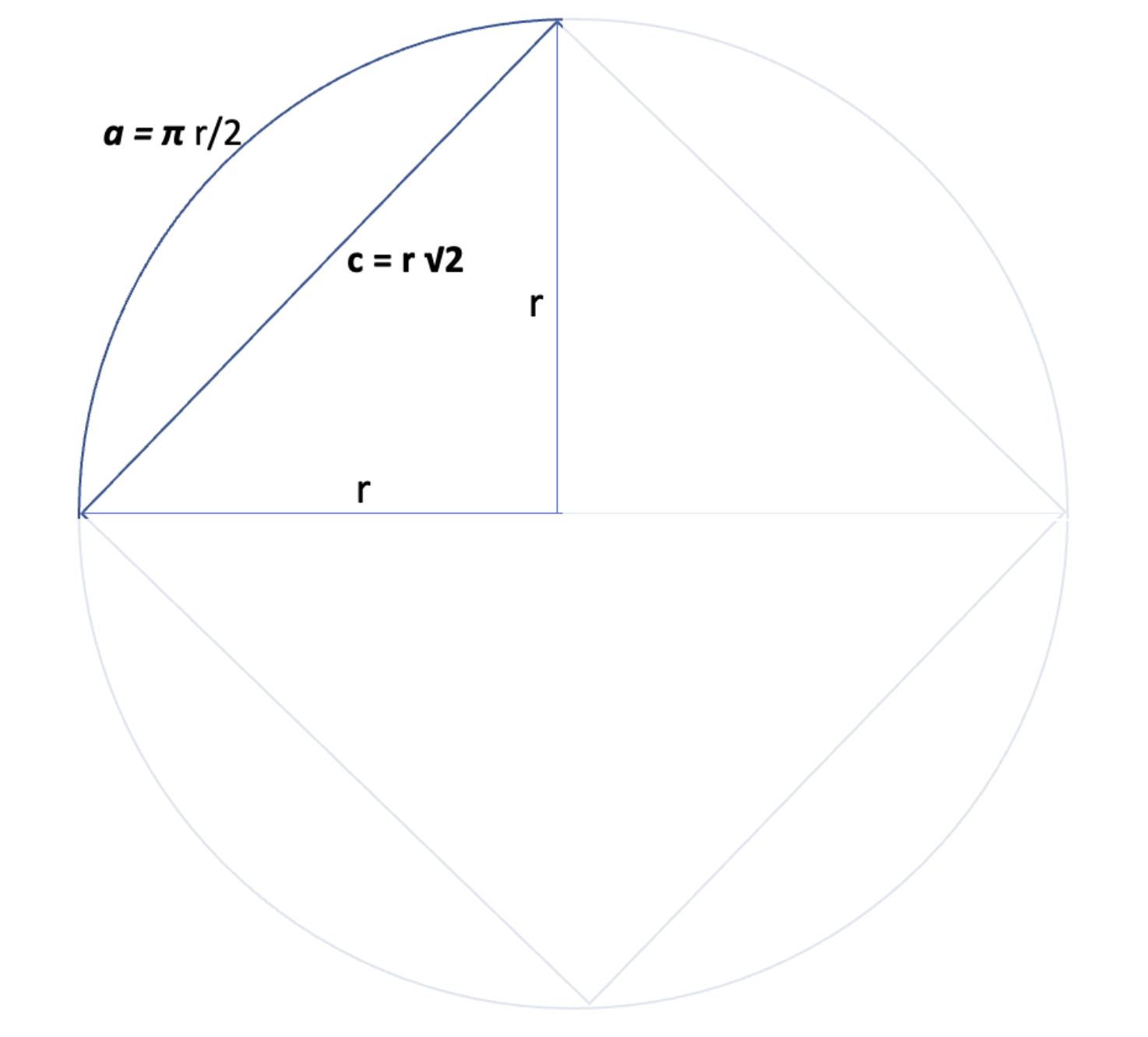Company and Culture

2022-07-22

# 22/7 is not 𝝅 (but maybe 16/5 is)

## We celebrate 𝝅 on 14/3 (March 14th which is by the way also the birthday of Albert Einstein) because if you write it 3/14 you have the first three digits of 𝝅.

We celebrate 𝝅 on 14/3 (March 14th which is by the way also the birthday of Albert Einstein) because if you write it 3/14 you have the first three digits of 𝝅.
But we also celebrate it on 22/7 (July 22nd) because 22/7 (22 divided by 7) is approx. 3,14. And we celebrate it on 28/6 (June 28th) because 6,28 is an approximation of 2*𝝅 (or tau).
But maybe we should forget all these celebration dates and celebrate 𝝅 on 16/5 (May 5th which is by the way also the death-day of Joseph Fourier) because if you divide 16 by 5 you get 3,2 which is the real value of 𝝅. Forget about all the hype, the allegedly transcendence and infinity of 𝝅.
𝝅 is really just 3,2.
Don’t believe me? It’s quite simple to prove and there is even a legislative process pending in the US to define 𝝅 as 3,2 (once and for all, at least in Indiana). So here is the story:

## Why 𝝅 is (also) 3,2

Edward Johnston Goodwin, a hobby mathematician, discovered 1888 in a supernatural manner the exact measurements of a circle. Afterward he began to work on squaring the circle. In 1894 he published the “correct way” of squaring the circle in his article “Quadrature of the circle” appeared in the first edition of the mathematical journal “American Mathematical Monthly”. It turn out that the journal was not proof reading (or even peer reviewing) his article because they printed it as a filler on the own request of Goodwin with a disclaimer saying, “published by request of the author”.
Goodwin used the below model circle which has a radius of r.The arc (a) is 𝝅 * r/2 (because the complete circumference is 𝝅 * 2r) where 𝝅 is the variable we want to resolve. The chord (c) is r * √2.
Goodwin now claims that “the ratio of the chord (c) and arc (a) is 7:8”. Goodwin assumed √2 to be 10/7 because he claimed “the ratio of the diagonal and one side of a square which is as ten to seven“. With this we can calculate 𝝅 to be 16/5 or 3,2.
When we calculate with √2 we get:
``````The ratio of the chord (c) and arc (a) is 7:8, with c = r * √ 2 and a = 𝝅 * r/2.

7/8 = (r * √2)/(𝝅 * r/2)
7/8 = (r * √2)/(𝝅 * r/2)
7/8 = (2√2)/𝝅
8/7 = 𝝅/(2√2)
(8*/(2√2))/7 = 𝝅
(16/7)√2 = 𝝅 = 3,2
``````
To make the confusion even more perfect his article says (at the very beginning) something like “the surface area of a circle is equal to the square over a line segment which is equal to a quarter of the circumference”. In other words, this would mean that 𝝅 (which is defined as the ratio of a circle’s circumference to its diameter) would be 4 (or even be 0) in this case. It seems that it possible to read Goodwin’s article in such different ways that 𝝅 can have more than one possible value. Later he committed himself to an interpretation which favored 3,2.
Now you probably think: Wow, that’s crazy. Luckily no one took this seriously.
But wait, it gets even more crazier.

## The “Indiana PI Bill”

He put his result under copyright and went to the state representative of Indiana, Taylor I. Record, to suggest a bill „introducing a new mathematical truth“ to be introduced in the Indiana House of Representatives. And so, Record did. This bill, called the “Indiana PI Bill” does indeed not mention 𝝅 at all but says that “The ratio of the diameter and circumference [of a circle] is as five-fourths to four …” which would mean that 𝝅 is 3,2.
The house passed the bill in February 1897 without a dissenting vote. You may now ask: Why did they so? Is just that easy that Goodwin offered the state of Indiana a free license to use his “new mathematical truth” for free, while others need to pay for it (because he holds the copyright and planned to charge people using his “discoveries”). So, no one at the House did question the merits of the bill and took it’s mathematical correctness for granted because the “American Mathematical Monthly” has published it, didn’t they?.
The chance to get it for free was too compelling. The complete title of the bill was, “A Bill for an act introducing a new mathematical truth and offered as a contribution to education to be used only by the State of Indiana free of cost by paying any royalties whatever on the same, provided it is accepted and adopted by the official action of the Legislature of 1897”, and so it seems they feared that if they would not pass the bill the state of Indiana have to pay for it.
Thankfully now people began to question Goodwins calculations, and after an outcry of the mathematical society, Purdue University Professor C. A. Waldo talked to the senators of Indiana trying to clarify the mathematical substance of the bill. In Indiana the Senate as the second chamber after the House need to pass a bill to sign it into law. The Senate had a session and postponed the bill indefinitely. But not because the senators start to question the merits of the bill. No, they postponed the bill because one senator observed that the General Assembly lacked the power to define mathematical truth in general.
Howsoever, lucky people of Indiana - would have been interesting to see what have happened in the 20th and 21st century when people were forced to calculate building and factories in Indiana using a value of 3,2 for 𝝅.

## Why Goodwin was wrong?

That’s quite obvious, isn’t it?
We know that if you multiply six by nine you get 42 (that’s the missing fact that Goodwin wasn’t aware of in 1894 for evident reasons).
``````6*9 = 42
``````
That would mean
``````7*9 = 51
9*7 = 51
8*7 = 44
7*8 = 44
7/8 = 44/64 = 11/16
``````
``````Again the ratio of the chord (c) and arc (a) is 7:8, with c = r * √ 2 and a = 𝝅 * r/2.

7/8 = 11/16 = (r * √2)/(𝝅 * r/2)
11/16 = (r * √2)/(𝝅 * r/2)
11/16= (2√2)/𝝅
16/11= 𝝅/(2√2)
(16*/(2√2))/11 = 𝝅
(32/11)√2 = 𝝅 = 4,11 ...
``````

## Conclusion

If 𝝅 is not 4,11 (which it is not, it is 3,1415926535 …) something must be fundamentally wrong with the universe
q.e.d
So we must again move the 𝝅 celebration day to, let’s say, 11th of April (4,11) which is, by mere coincidence, the birthday of Sir Andrew Wiles (a English mathematician known for proving Fermat’s Last Theorem).
References:
https://www.wrtv.com/news/local-news/indianapolis/it-would-have-been-a-laughingstock-hoosier-lawmakers-nearly-passed-the-indiana-pi-bill-in-1897
http://www.indianalegalarchive.com/journal/2015/3/14/legislating-pi
https://en.wikipedia.org/wiki/Pi
https://en.wikipedia.org/wiki/Indiana_Pi_Bill
https://en.wikipedia.org/wiki/Andrew_Wiles
http://www.6by9.net/why-6by9/
Interested? Get in touch!
Drop us a note and we get in contact.